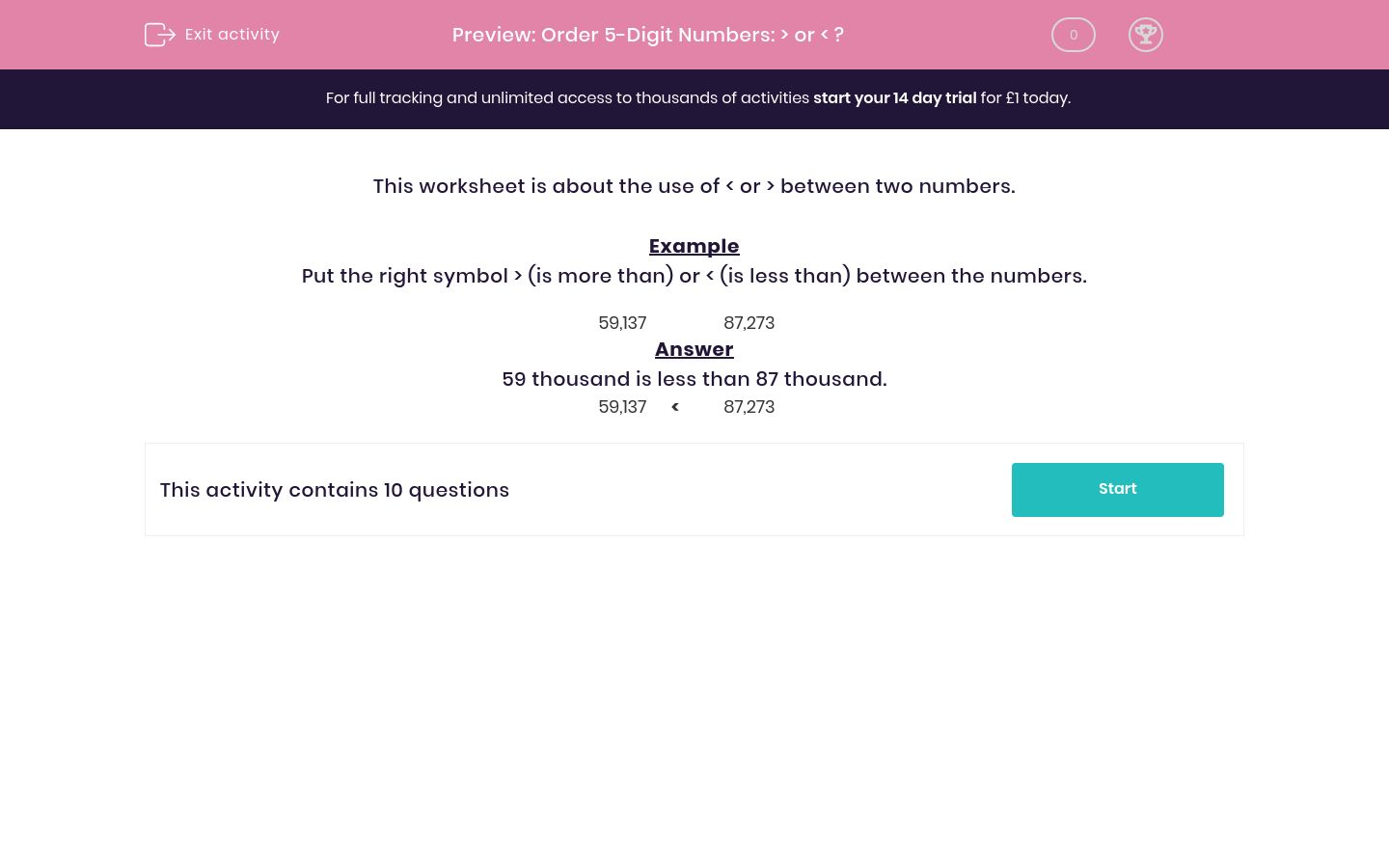# Order 5-Digit Numbers: > or < ?

In this worksheet, students use the symbols > and < correctly between two large numbers.Key stage:  KS 2

Curriculum topic:   Maths and Numerical Reasoning

Curriculum subtopic:   Place Value

Difficulty level:### QUESTION 1 of 10

This worksheet is about the use of < or > between two numbers.

Example

Put the right symbol > (is more than) or < (is less than) between the numbers.

59,137    87,273

59 thousand is less than 87 thousand.

59,137
 <
87,273

Put the right symbol > (is more than) or < (is less than) between the numbers.

32,006    91,873
<

>

Put the right symbol > (is more than) or < (is less than) between the numbers.

92,563    32,287
<

>

Put the right symbol > (is more than) or < (is less than) between the numbers.

10,159    20,335
<

>

Put the right symbol > (is more than) or < (is less than) between the numbers.

60,974    95,509

<

>

Put the right symbol > (is more than) or < (is less than) between the numbers.

70,251    13,255

<

>

Put the right symbol > (is more than) or < (is less than) between the numbers.

25,556    84,782

<

>

Put the right symbol > (is more than) or < (is less than) between the numbers.

85,673    92,389

<

>

Put the right symbol > (is more than) or < (is less than) between the numbers.

83,132    47,328

<

>

Put the right symbol > (is more than) or < (is less than) between the numbers.

22,124    22,770

<

>

Put the right symbol > (is more than) or < (is less than) between the numbers.

59,137    59,173

<

>

• Question 1

Put the right symbol > (is more than) or < (is less than) between the numbers.

32,006    91,873
<
• Question 2

Put the right symbol > (is more than) or < (is less than) between the numbers.

92,563    32,287
>
• Question 3

Put the right symbol > (is more than) or < (is less than) between the numbers.

10,159    20,335
<
• Question 4

Put the right symbol > (is more than) or < (is less than) between the numbers.

60,974    95,509

<
• Question 5

Put the right symbol > (is more than) or < (is less than) between the numbers.

70,251    13,255

>
• Question 6

Put the right symbol > (is more than) or < (is less than) between the numbers.

25,556    84,782

<
• Question 7

Put the right symbol > (is more than) or < (is less than) between the numbers.

85,673    92,389

<
• Question 8

Put the right symbol > (is more than) or < (is less than) between the numbers.

83,132    47,328

>
• Question 9

Put the right symbol > (is more than) or < (is less than) between the numbers.

22,124    22,770

<
• Question 10

Put the right symbol > (is more than) or < (is less than) between the numbers.

59,137    59,173

<
---- OR ----

Sign up for a £1 trial so you can track and measure your child's progress on this activity.

### What is EdPlace?

We're your National Curriculum aligned online education content provider helping each child succeed in English, maths and science from year 1 to GCSE. With an EdPlace account you’ll be able to track and measure progress, helping each child achieve their best. We build confidence and attainment by personalising each child’s learning at a level that suits them.

Get started